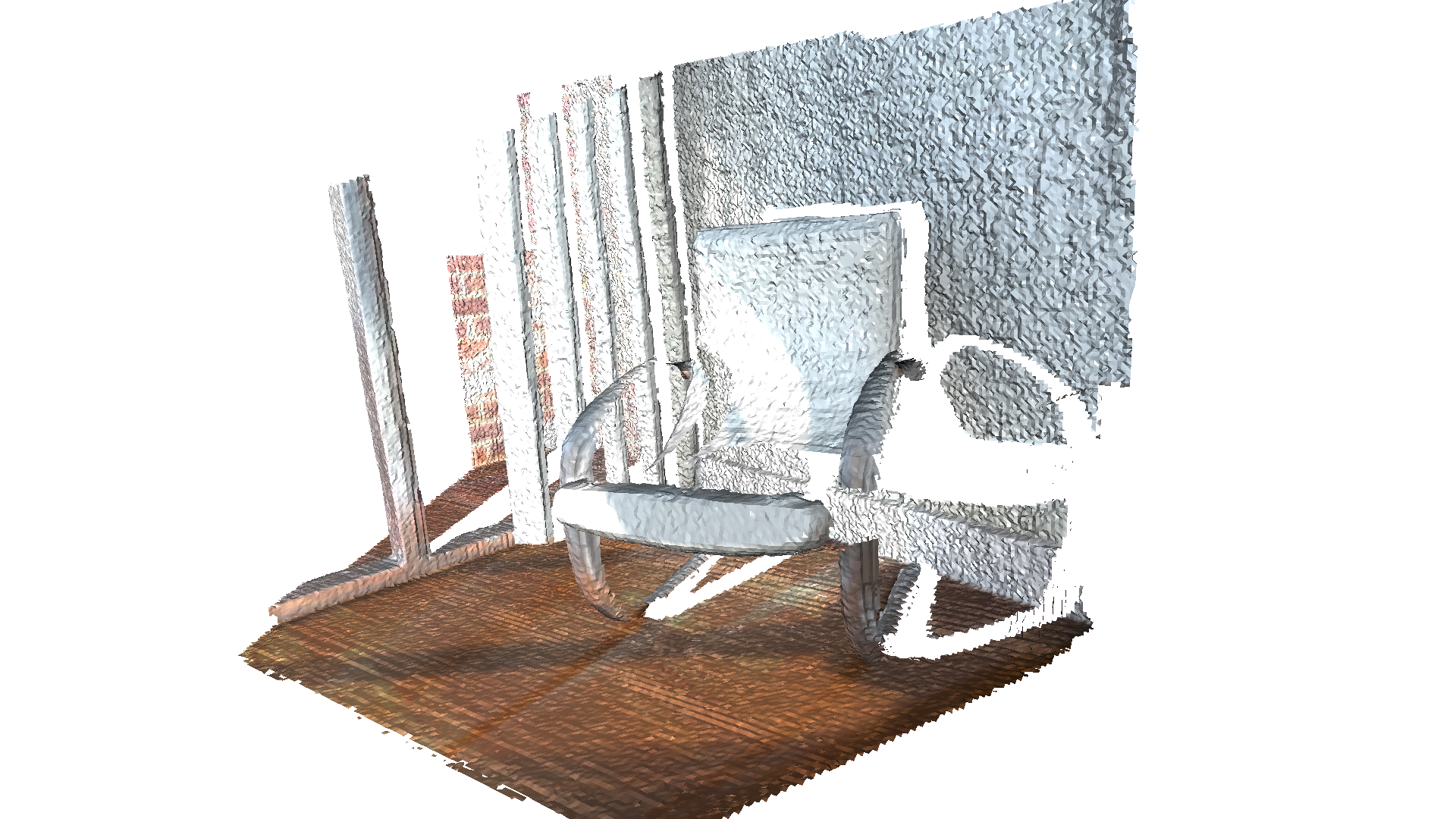# RGBD integration¶

Open3D implements a scalable RGBD image integration algorithm. The algorithm is based on the technique presented in [Curless1996] and [Newcombe2011]. In order to support large scenes, we use a hierarchical hashing structure introduced in Integrater in ElasticReconstruction.

## Read trajectory from .log file¶

This tutorial uses the function read_trajectory to read a camera trajectory from a .log file. A sample .log file is as follows.

# odometry.log
0   0   1
1   0   0   2
0   1   0   2
0   0   1 -0.3
0   0   0   1
1   1   2
0.999988  3.08668e-005  0.0049181  1.99962
-8.84184e-005  0.999932  0.0117022  1.97704
-0.0049174  -0.0117024  0.999919  -0.300486
0  0  0  1

:

class CameraPose:

def __init__(self, meta, mat):
self.pose = mat

def __str__(self):
return 'Metadata : ' + ' '.join(map(str, self.metadata)) + '\n' + \
"Pose : " + "\n" + np.array_str(self.pose)

traj = []
with open(filename, 'r') as f:
while metastr:
mat = np.zeros(shape=(4, 4))
for i in range(4):
mat[i, :] = np.fromstring(matstr, dtype=float, sep=' \t')
return traj

:

redwood_rgbd = o3d.data.SampleRedwoodRGBDImages()


## TSDF volume integration¶

Open3D provides two types of TSDF volumes: UniformTSDFVolume and ScalableTSDFVolume. The latter is recommended since it uses a hierarchical structure and thus supports larger scenes.

ScalableTSDFVolume has several parameters. voxel_length = 4.0 / 512.0 means a single voxel size for TSDF volume is $$\frac{4.0\mathrm{m}}{512.0} = 7.8125\mathrm{mm}$$. Lowering this value makes a high-resolution TSDF volume, but the integration result can be susceptible to depth noise. sdf_trunc = 0.04 specifies the truncation value for the signed distance function (SDF). When color_type = TSDFVolumeColorType.RGB8, 8 bit RGB color is also integrated as part of the TSDF volume. Float type intensity can be integrated with color_type = TSDFVolumeColorType.Gray32 and convert_rgb_to_intensity = True. The color integration is inspired by PCL.

:

volume = o3d.pipelines.integration.ScalableTSDFVolume(
voxel_length=4.0 / 512.0,
sdf_trunc=0.04,
color_type=o3d.pipelines.integration.TSDFVolumeColorType.RGB8)

for i in range(len(camera_poses)):
print("Integrate {:d}-th image into the volume.".format(i))
rgbd = o3d.geometry.RGBDImage.create_from_color_and_depth(
color, depth, depth_trunc=4.0, convert_rgb_to_intensity=False)
volume.integrate(
rgbd,
o3d.camera.PinholeCameraIntrinsic(
o3d.camera.PinholeCameraIntrinsicParameters.PrimeSenseDefault),
np.linalg.inv(camera_poses[i].pose))

Integrate 0-th image into the volume.
Integrate 1-th image into the volume.
Integrate 2-th image into the volume.
Integrate 3-th image into the volume.
Integrate 4-th image into the volume.


## Extract a mesh¶

Mesh extraction uses the marching cubes algorithm [LorensenAndCline1987].

:

print("Extract a triangle mesh from the volume and visualize it.")
mesh = volume.extract_triangle_mesh()
mesh.compute_vertex_normals()
o3d.visualization.draw_geometries([mesh],
front=[0.5297, -0.1873, -0.8272],
lookat=[2.0712, 2.0312, 1.7251],
up=[-0.0558, -0.9809, 0.1864],
zoom=0.47)

Extract a triangle mesh from the volume and visualize it.Note:

TSDF volume works like a weighted average filter in 3D space. If more frames are integrated, the volume produces a smoother and nicer mesh. Please check Make fragments for more examples.